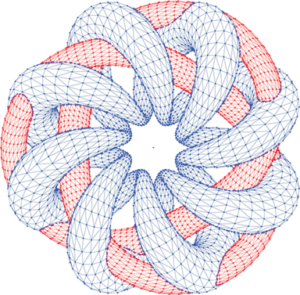# Robust, Generic, and Efficient Construction of Envelopes of Surfaces in Three-Dimensional Space

## AbstractThe minimization diagram of two triangulated surfaces with approximately 16,000 triangles. The triangulated surfaces input files were taken from here

Lower envelopes are fundamental structures in computational geometry that have many applications, such as computing general Voronoi diagrams and performing hidden surface removal in computer graphics. We present a generic, robust and efficient implementation for computing the envelopes of surfaces in3. To the best of our knowledge, this is the first exact implementation that computes envelopes in three-dimensional space. Our implementation is based on CGAL and is designated as a CGAL package. The separation of topology and geometry in our solution allows the reuse of the algorithm with different families of surfaces, provided that a small set of geometric objects and operations on them is supplied. We used our algorithm to compute the lower and upper envelope for several types of surfaces. Our implementation follows the exact geometric computation paradigm. Since exact arithmetic is typically slower than floating-point arithmetic, especially when higher order surfaces are involved, we minimize the number of such operations, to gain better performance in practice. Our experiments show interesting phenomena in the behavior of the divide-and-conquer algorithm and the combinatorics of lower envelopes of random surfaces.

• Michal Meyerovitch
Robust, Generic, and Efficient Construction of Envelopes of Surfaces in Three-Dimensional Space
In Proceedings of the 14th Annual European Symposium on Algorithms (ESA), pages 792-803, Zurich, Switzerland, 2006 [link] [bibtex]
• Michal Meyerovitch
Robust, generic and efficient construction of envelopes of surfaces in three-dimensional space
M.Sc. thesis [pdf] [bibtex]
• The code is available as a part of CGAL. The documentation of the package can be found in this link.
• Some input sets (for triangles and spheres)

### Contacts

Michal Meyerovitch